### Home > CALC > Chapter 5 > Lesson 5.2.2 > Problem5-72

5-72.
1. Evaluate the following integrals without a calculator. Homework Help ✎1.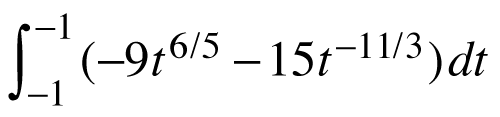2.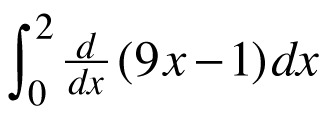3.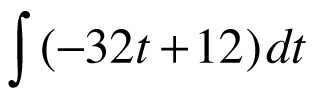4.5.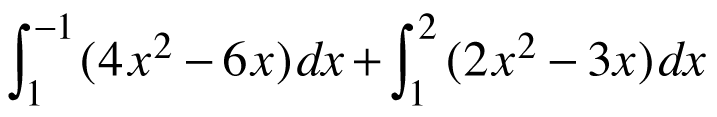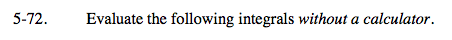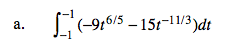Fundamental Theorem of Calculus, Part 2.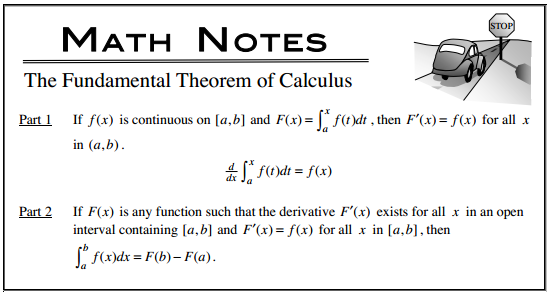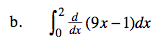Fundamental Theorem of Calculus, Part 1.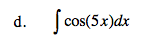ksin(5x) + C
Find k.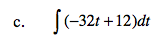−16t ² + 12t + C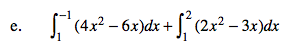$\frac{4}{3}x^3-3x^2\Big|_1^{-1}-\frac{2}{3}x^3-\frac{3}{2}x^2\Big|_1^2=-\frac{7}{3}$